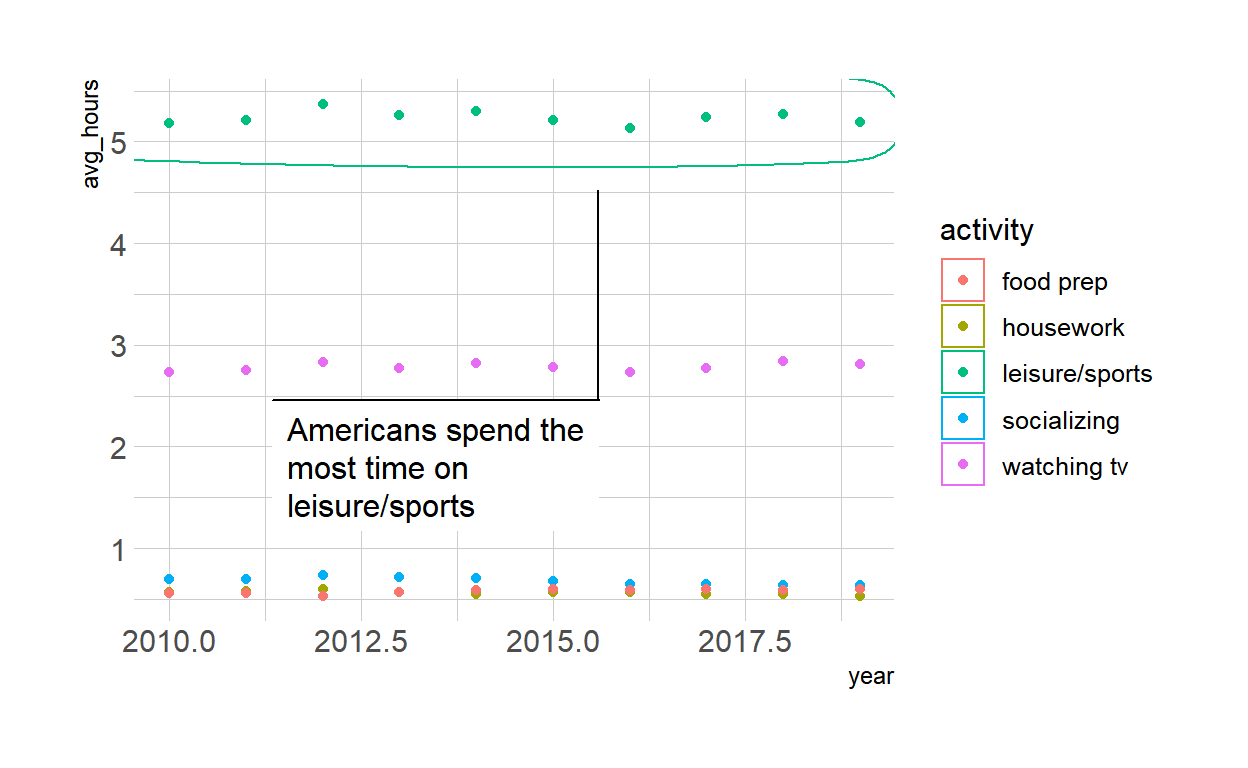# Data Visualization

Code for quiz 9

1. Load the R packages we will use
``````library(tidyverse)
library(echarts4r)
library(ggforce)
library(hrbrthemes)
library(tidyquant)
theme_set(theme_ipsum())
``````

# Question: e_charts-1

• Create a bar chart that shows the average hours Americans spend on five activities by year.

• Use `timeline` argument to create an animation that will animate through the years.

• Read it into `spend_time`

``````spend_time <- read_csv("spend_time.csv")
``````
• Start with `spend_time`

• Then group_by `year`

• Then create an e_chart that assigns `activity` to the x-axis and will show activity by year.

• Then use `e_timeline_opts` to set autoPlay to TRUE

• Then use `e_bar` to represent the variable `avg_hours` with a bar chart

• Then use `e_title` to set the main title to ‘Average hours Americans spend per day on each activity’

• Then remove the legend with `e_legend`

``````spend_time %>%
group_by(year)  %>%
e_charts(x= activity, timeline = TRUE)  %>%
e_timeline_opts(autoPlay = TRUE) %>%
e_bar(serie = avg_hours)  %>%
e_title(text = "Average hours Americans spend per day on each activity") %>%
e_legend(show = FALSE)
``````

# Question: echarts-2

• Create a line chart for activities that American spend time on.

• Start with `spend_time`

• Then use `mutate` to convert `year` from a number to a string (year-month-day) using `mutate`

• First convert `year` to a string “201x-12-31” using function `paste`

• `paste` will paste each year to 12 and 31 (separated by -) THEN

• Use `mutate` to convert year from character object to a date object using `ymd` from the `lubridate` package.

• `ymd` function converts dates stored as characters to date objects

• Then `group_by` the variable `activity` (to get a line for each activity)

• Then initiate an `e_charts` object with `year`on the x-axis

• Then use `e_line` to add a line to the variable `avg_hours`

• Then add tooltip with `e_tooltip`

• Then use `e_title` to set the main title ‘Average hours Americans spend per day on each activity’

• Then use `e_legend (top = 40)` to move the legend down (from the top)

``````spend_time %>%
mutate(year = paste(year, "12", "31", sep = "-")) %>%
mutate(year = lubridate::ymd(year)) %>%
group_by(activity) %>%
e_charts(x = year) %>%
e_line(serie = avg_hours) %>%
e_tooltip() %>%
e_title(text = "Average hours Americans spend per day on each activity") %>%
e_legend(top = 40)
``````

# Question: Modify Slide 82

• Create a plot with `spend_time` data

• Assign `year` to the x-axis

• Assign `avg_hours` to the y-axis

• Assign `activity` to color

• Add points with `geom_point`

• Add `geom_mark_ellipse`

• Filter on activity == “leisure/sports”

• Description: “Americans spend the most time on leisure/sports”

``````ggplot(spend_time, aes(x = year, y = avg_hours, color = activity)) +
geom_point() +
geom_mark_ellipse(aes(filter = activity == "leisure/sports",
description = "Americans spend the most time on leisure/sports"))
``````# Question: tidyquant

• Retrieve stock price for Google, ticker: GOOG using `tg_get`

• From 2019-08-01 to 2020-07-28

• Assign the output to `df`

``````df <- tq_get("GOOG", get = "stock.prices", from = "2019-08-01", to = "2020-07-28")
``````

• Create a plot with `df` data

• Assign `date` to the x-axis

• Assign `close` to the y-axis

• Add a line with `geom_line`

• Add `geom_mark_ellipse`

• Filter on a date to mark, pick a date after looking at the line plot.

• Include the date in your Rmd code chunk

• Include a description of something that happened on that date from the pandemic timeline

• Include the description in your Rmd code chunk

• Fill the ellipse yellow

• Add `geom_mark_ellipse`

• Filter on a date that had minimum `close` price

• Include the date in your Rmd code chunk

• Include a description of something that happened on that date from the pandemic timeline

• Include the description in your Rmd code chunk

• Color the ellipse red

• Add `labs`

``````ggplot(df, aes(x = date, y = close)) +
geom_line() +
geom_mark_ellipse(aes(
filter = date == "2020-04-14",
description = "Trump announced decision to halt funding to WHO"),
fill = "yellow") +
geom_mark_ellipse(aes(
filter = date == "2020-07-17",
description = "U.S. records highest single day infections"), color = "red") +``````ggsave(filename = "preview.png", path = here::here("_posts", "2022-04-01-data-visualization"))